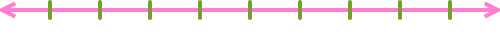# 7th Grade Mathematics Quiz! Can You Pass This Test?

20 Questions | Total Attempts: 575SettingsBelow is a 7th grade mathematics quiz, can you pass this test? There are different ways that a person can polish up their math skills and one of the most common is having regular exercises for questions they have a hard time answering. By tackling this quiz you will get to see which math problems are problematic for you. Do check them out and take it as many times as you need.

• 1.
Find the next three terms in the sequence: 1, 1, 2, 4, 7, 13, 24
• A.

3, 40, 67

• B.

44, 81, 149

• C.

13, 7, 4

• D.

37, 61, 98

• 2.
What represents (-4)?
• A.

(+)(+)(-)

• B.

(-)(-)(+)(+)

• C.

(+)(-)(+)(-)(-)(-)(-)(-)

• D.

(-)(+)(-)(-)(-)

• 3.
What is 43?
• A.

64

• B.

16

• C.

4

• D.

20

• 4.
Last year Jan had 25 model airplanes in her collection. This year she has 32% more model airplanes. Jan then gave her brother 6 model airplanes to start a collection. How many model airplanes does Jan have after she gave 6 to her brother?
• A.

8

• B.

27

• C.

33

• D.

51

• 5.
Calculate: (-8) + (+2) - (-6) = ?
• A.

16

• B.

0

• C.

4

• D.

3

• 6.
Which equation is true?
• A.

5/8 = -5/8

• B.

5/8 = -(-5/-8)

• C.

-5/8=4/8

• D.

-3/-4= -3/4

• 7.
What is the percentage of 3/20?
• A.

15 %

• B.

34 %

• C.

3 %

• D.

20 %

• 8.
Write the prime factorization of 6. Use exponents when appropriate and order the factors from least to greatest (for example,  2 2 3 5 ).
• A.

2*3

• B.

2^2^2

• C.

3*2

• D.

2^4

• 9.
What is the greatest common factor of 20 and 17?
• A.

1

• B.

340

• C.

2

• D.

10

• 10.
Type the missing number : -4,-3,-2,-1,0,1__,3,4
• A.

2

• B.

3

• C.

6

• D.

8

• 11.
A glass vase factory recently produced 2 vases that were chipped and 8 vases that were not. Considering this data, how many of the next 20 vases would you expect to be chipped?
• A.

4

• B.

8

• C.

16

• D.

32

• 12.
What is |-9|?
• A.

-9

• B.

9

• C.

90

• D.

.09

• 13.
Jackie, a florist, is assembling bouquets of roses to sell at her flower shop. The number of bouquets she can assemble depends on how many roses are in her shop. b = the number of bouquets Jackie can assembler = the number of roses in the flower shop Which of the variables is independent and which is dependent?
• A.

B is the independent variable and r is the dependent

• B.

R is the independent variable and b is the dependent variable

• C.

Both are dependent

• D.

Both are independent

• 14.
Evaluate:4! =
• A.

34

• B.

14

• C.

24

• D.

240

• 15.
Which property of multiplication is shown?   9 × (1 × 4) = (9 × 1) × 4
• A.

Commutative

• B.

Distributive

• C.

Identity

• D.

Associative

• 16.
Look at this set of 8 numbers: 28   52   44   28   11   57   15   61How would the mean change if the number 41 replaced the number 57 in the set?
• A.

Decrease

• B.

Increase

• C.

No change

• D.

Both have increased and decreased

• 17.
What is the length of the hypotenuse , if the length is 3km and the base is 4 km .
• A.

10 km

• B.

20 km

• C.

30 km

• D.

40 km

• 18.
Does the point(7, 1) satisfy the equation y = x – 6?
• A.

No

• B.

Yes

• 19.
A cookie factory produced 803 packages of cookies. There were 82 cookies in each package. About how many cookies did the factory produce in all? Choose a better estimate.
• A.

65846

• B.

65879

• C.

65864

• D.

6789

• 20.
A miniature golf course recently provided its customers with 12 golf balls, of which 4 were orange. Based on experimental probability, how many of the next 18 golf balls handed out should you expect to be orange golf balls?
• A.

6

• B.

5

• C.

7

• D.

8

Related TopicsBack to top